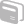# Activities

••• ##### Subject Area

• Math: Algebra I: Equations and Inequalities
• Math: Algebra I: Expressions
• Math: Algebra I: Linear Functions
• Math: Algebra I: Functions and Relations

• ##### Author9-12

1 Hours

• ##### Device
• TI-Navigator™
• ##### Software

LearningCheck™ Creator

## First Semester Algebra Basics

#### Activity Overview

Learn check file that includes problems that require the student to: 1)Evaluate expressions 2)Classify properties 3)Define domain, range, relation, function, independent, dependent 4)Solve equations 5)Classify the quadrants 6)Locate points 7)Determine if pictures are functions 8)Determine domain, range 9)Match graphs with equations or characteristics 10)Determine how many points of intersection 11)Classify graphs of slopes 12)Determine slope from two points 13)Determine slope from standard form.

#### Before the Activity

Learn check file that includes problems that require the student to: 1)Evaluate expressions 2)Classify properties 3)Define domain, range, relation, function, independent, dependent 4)Solve equations 5)Classify the quadrants 6)Locate points 7)Determine if pictures are functions 8)Determine domain, range 9)Match graphs with equations or characteristics 10)Determine how many points of intersection 11)Classify graphs of slopes 12)Determine slope from two points 13)Determine slope from standard form.

#### During the Activity

Students will take the Learning Check to demonstrate their understanding of the concepts. The teacher should collect the results via the TI-Navigator

#### After the Activity

The teacher will be able to gauge student strenghts and weaknesses on these concepts after students have submitted their Learning Check via te TI Navigator.